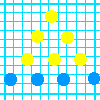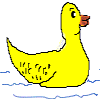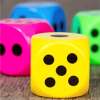#### You may also like### Graphing Number Patterns

Does a graph of the triangular numbers cross a graph of the six times table? If so, where? Will a graph of the square numbers cross the times table too?### Dining Ducks

Use the information about the ducks on a particular farm to find out which of the statements about them must be true.### You Never Get a Six

Charlie thinks that a six comes up less often than the other numbers on the dice. Have a look at the results of the test his class did to see if he was right.

# How Big Are Classes 5, 6 and 7?

##### Age 7 to 11Challenge Level

Mikey from Archibishop of York C of E Junior School thought hard about this problem. He says:

Using the bar graph for class $4$ gives $36$ pupils but this allows several possible answers:
$5\times4 + 4\times4 = 36$ but so does
$6\times4 + 3\times4$,
$7\times4 + 2\times4$,
$8\times4 + 1\times4$.
All these are the same as $9\times4$. We know big plus small must equal $9$ but not the split from the info given. Maybe if Katie and Charlene had done classes $1$ to $3$ as well we would have been able to work it out.

Well done, Mikey for seeing that there are several possible solutions - not many of you realised this. What answers are possible then? Might some be more likely than others do you think?

Lizzi from Bampton C of E Primary School wrote:

By looking at the amount of pupils in class $4$ on the bar graph, you can tell that the big people on the graph equal $6$ and the little people equal $3$ people, so therefore there are $42$ people in class $7$, $39$ people in class $6$ and $30$ people in class $5$ which equals $111$ pupils.

This is certainly one of the possibilities. Freddie from Whitehall Primary School calculated another one:

Big people = $7$
Small people = $2$
Year 5 = $35$ Year 6 = $38$ Year 7 = $40$
Total = $113$

Joshua from BMGS suggests:

... for each big figure it was $8$ and for each small one it was $1$. Then it was a simple solution of adding up all of years $5, 6$ and $7$. The total answer was $115$.

We are still left without the solution for a big stickman representing $5$ children and a small stickman representing $4$ children, but thank you for all your contributions .